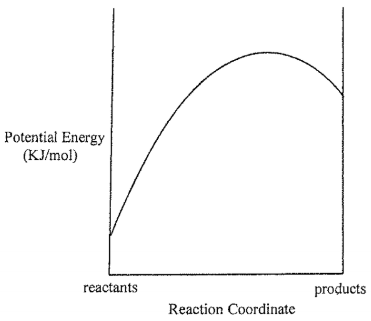# Problem: Consider the Potential Energy vs. Reaction Coordinate diagram shown to the right. For each of the following questions, circle the best answer.If the temperature is lowered, i) equilibrium will shift toward reactantsii) equilibrium will shift toward productsiii) no change in equilibrium position

###### FREE Expert Solution

For this problem, we have to determine which among the statement is true when the temperature is lowered

• According to Le Chatelier’s Principle, if a system (chemical reaction) is at equilibrium and we disturb it, then the system will readjust to maintain its equilibrium state.
• In this case, we can estimate the sign of ΔH by using the reaction diagram provided###### Problem Details

Consider the Potential Energy vs. Reaction Coordinate diagram shown to the right. For each of the following questions, circle the best answer.If the temperature is lowered,

i) equilibrium will shift toward reactants

ii) equilibrium will shift toward products

iii) no change in equilibrium position